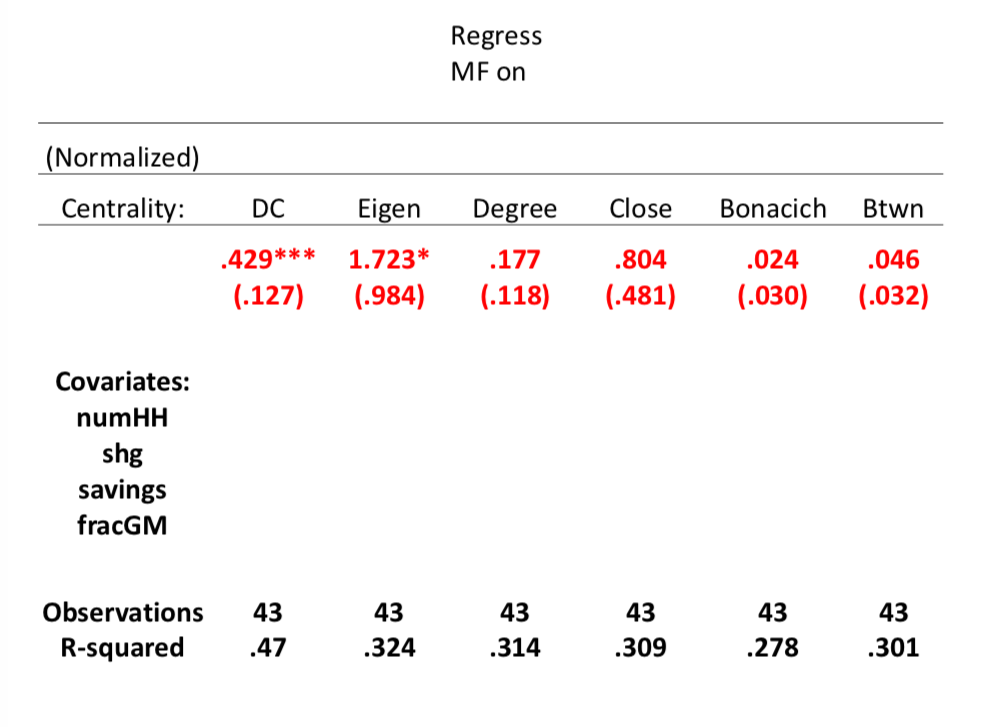# Diffusion Centrality: $DC_i(p, T)$¶

How many nodes are informed if:

• $i$ is initially informed

• each informed node tells each of its neighbors with prob $p$ in each period

• run for $T$ periods?

$$DC(p, T) = \sum_{t=1...T} (pg)^t 1$$

• $g$: adjacency matrix
• multiplies by 1, so that keep track of how many people we have heard at each point in time
• if $T=1:$ proportional to degree centrality, it just calculating how many people do I reach directly
• if $p < \frac{1}{\lambda_1}$ and $T$ is large, it will converge to Katz-Bonacich centrality, where $p$ plays the role of weight and $\lambda_1$ is the largest eigenvalue of the adjacency matrix
• if $p < \frac{1}{\lambda_1}$ and $T$ is large, it will approximate eigenvector centrality
• if $T$ is larege, becomes Bonacich centrality

Let's have a look at what this does in the India data that talk about beforeDC: diffusion centrality, we can see it have ***, so it is significant at 99% level, diffusion centrality here is defined by setting $p = \frac{1}{\lambda_1}$, then running it for some number of periods which was actually equivalent to the number of trimesters that a village had been exposed to information. So, some villages had 8 trimesters, some had 3 and so forth, but that gave us some numbers of rounds of communication that might go on in the village.

In order to figure out which one might be doing better explanation, we can sort of put them together in the same regressions, where we keep track of, of you know, things like the degree central of the leaders, the number of households, self-help group, participation savings fraction in the cast and so forth. So, if we keep track of all these controls, then if we do the diffusion centrality it turns out to be significant, eigenvector centrality is also significant. When we put them together, then the diffusion centrality remains highly significant and, and doesn't change much. The eigenvector centrality part of it is disappearing there.of it is disappearing there. And the idea here is diffusion centrality is keeping track of the fact that communication is not going on forever, it's only going on some finite number of times. And if you have some feeling for that, then this is a very practical measure which is designed to actually figure out how nodes are, what's they're position in a network as designed to spread information, and that particular measure seems to do very well in this sense. So, once we've looked at a very specific process, that can also suggest how we should measure the importance of nodes. We can weigh them directly by the process that we think might be governing the communication or whatever it is that we're examining in a particular network and that will give us a new idea of centrality measures or other kinds of measures of nodes. So, that's a little more about position in networks. There's a lot of ongoing research in this area, it's a fascinating area for study. but now, what we're going to turn to is, is beginning to understand network formation processes.